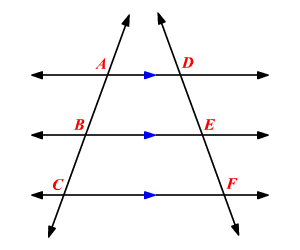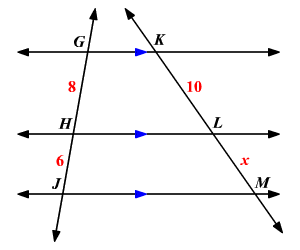# Parallel Lines and Proportionality

In the Triangle Proportionality Theorem , we have seen that parallel lines cut the sides of a triangle into proportional parts. Similarly, three or more parallel lines also separate transversals into proportional parts.If three or more parallel lines intersect two transversals, then they cut off the transversals proportionally.

In the figure shown, if $\stackrel{↔}{AD}\parallel \stackrel{↔}{BE}\parallel \stackrel{↔}{CF}$ , then $\frac{AB}{BC}=\frac{DE}{EF},\frac{AC}{DF}=\frac{BC}{EF}$ , and $\frac{AC}{BC}=\frac{DF}{EF}$ .

Example:

Find the value of $x$ .If three or more parallel lines intersect two transversals, then they cut off the transversals proportionally.

So write a proportion .

$\frac{GH}{HJ}=\frac{KL}{LM}$

Substitute the values.

$\frac{8}{6}=\frac{10}{x}$

Use the cross product .

$\begin{array}{l}\left(8\right)\left(x\right)=\left(10\right)\left(6\right)\\ \text{\hspace{0.17em}}\text{\hspace{0.17em}}\text{\hspace{0.17em}}\text{\hspace{0.17em}}\text{\hspace{0.17em}}\text{\hspace{0.17em}}\text{\hspace{0.17em}}\text{\hspace{0.17em}}8x=60\end{array}$

Divide each side by $8$ .

$\begin{array}{l}\frac{8x}{8}=\frac{60}{8}\\ \text{\hspace{0.17em}}\text{\hspace{0.17em}}\text{\hspace{0.17em}}x=7.5\end{array}$

Therefore, the value of $x$ is $7.5$ .